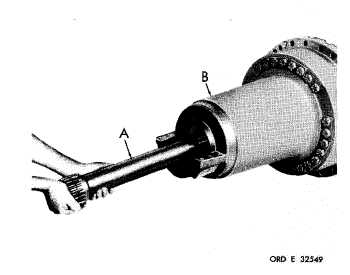Custom SearchP A R    76,   S T E P S   2 - 5 D I S A S S E M B L Y C H A P   5,   SEC   II Figure   130   (Step   2) R e m o v e    t h e    i n p u t    s h a f t    ( A )    f r o m    l e f t - o u t p ut d r i v e    a s s e m b l y    ( B ). Figure   132   (Step   4) Using   sling   (A)   and   two   3/8-24   x   1   bolts   (B) a n d    f l a t    w a s h e r s ,    p l a c e    t h e    a s s e m b l y    i n    an improvised    stand    (C). Figure   131   (Step   3) Using   lifting   eye   (A)   and   sling   (B)   to   prevent the   drive   assembly   from   turning,   and   using   a 1-1/8-inch wrench, loosen 24 bolts (C). Figure   133   (Step   5) Remove   twenty-two   bolts   (A)   and   washers   (B), leaving   two   of   the   bolts   (C),   180   degrees   apart. R e m o v e    t h r e e    s c r e w s    ( D ). 1 01